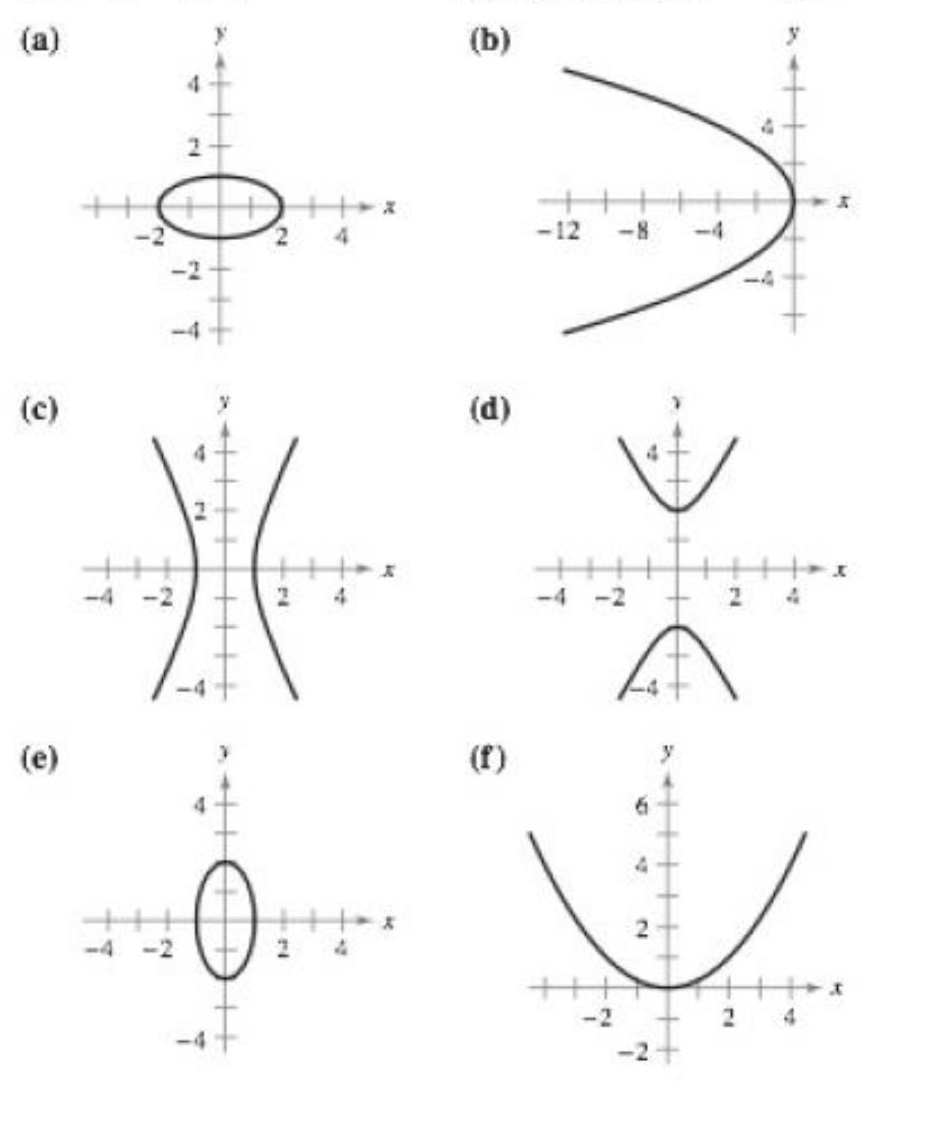Chapter 10, Problem 4RE

Chapter
Section
Textbook Problem

# Matching In Exercises 1-6, match the equation with its graph. [The graphs are labeled (a), (b), (c), (d), (e), and (f).]y 2   −   4 x 2   =   4

To determine

If the given equation y24x2=4 matches with its graph.

Explanation

Given: y24x2=4

The graphs are:

Refer to figure in question.

Consider the given equation:

y24x2=4

Here, x and y both are squared and one of them has a negative coefficient. Thus, we can conclude that this equation represents a hyperbola.

In order to find whether this hyperbola has a vertical or horizontal axis, present the given equation in the standard form.

Divide throughout by 4

14y2x2=1

Rewrite in the standard form as

(

### Still sussing out bartleby?

Check out a sample textbook solution.

See a sample solution

#### The Solution to Your Study Problems

Bartleby provides explanations to thousands of textbook problems written by our experts, many with advanced degrees!

Get Started

#### Find more solutions based on key concepts